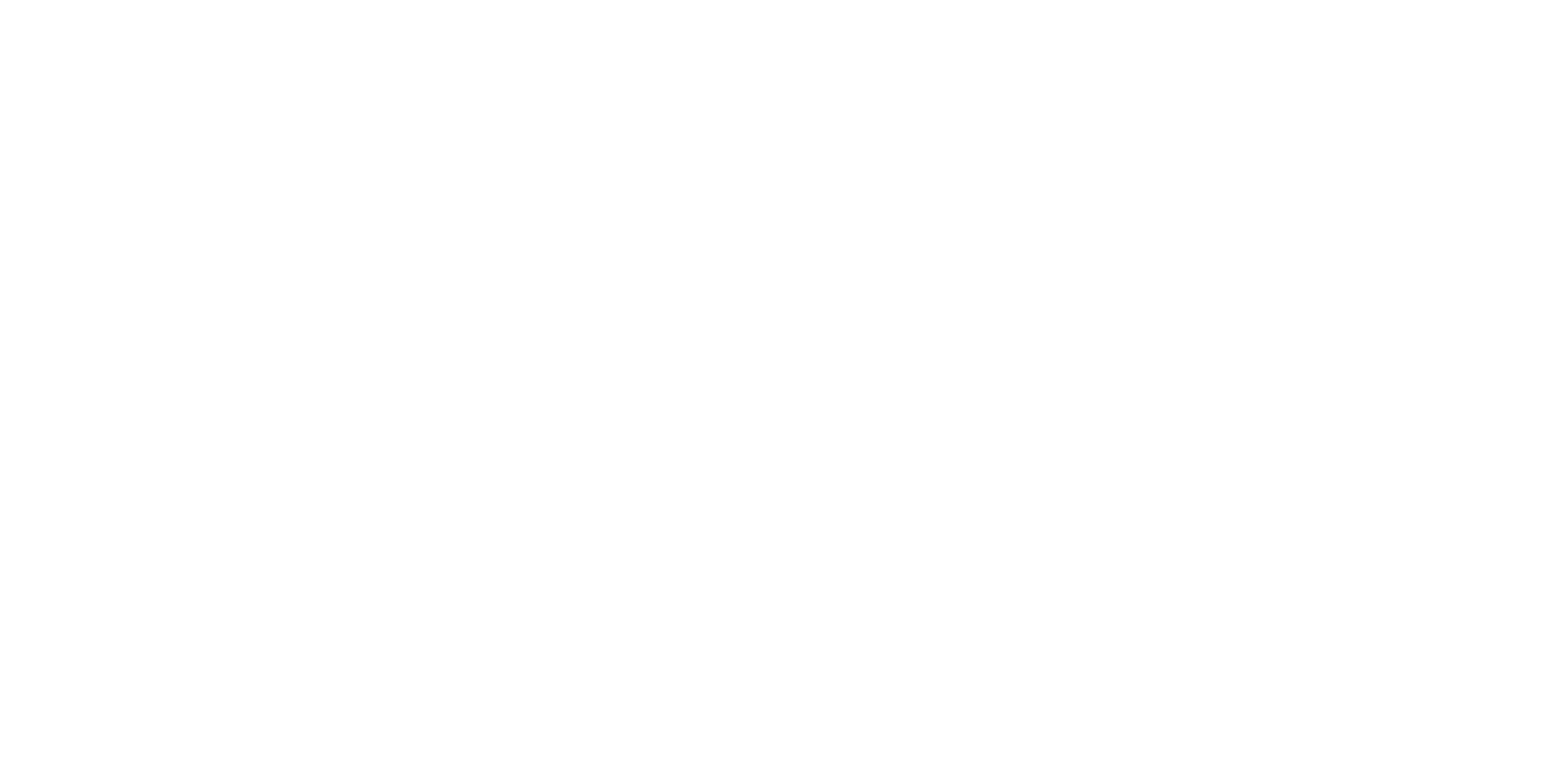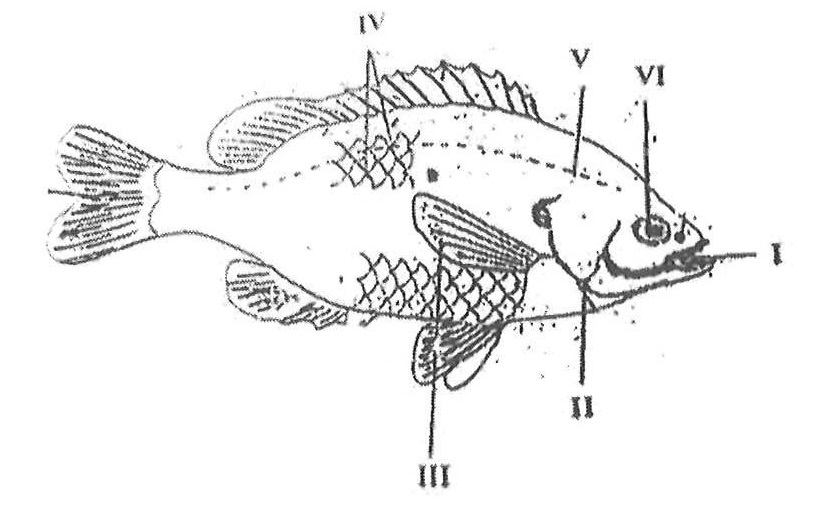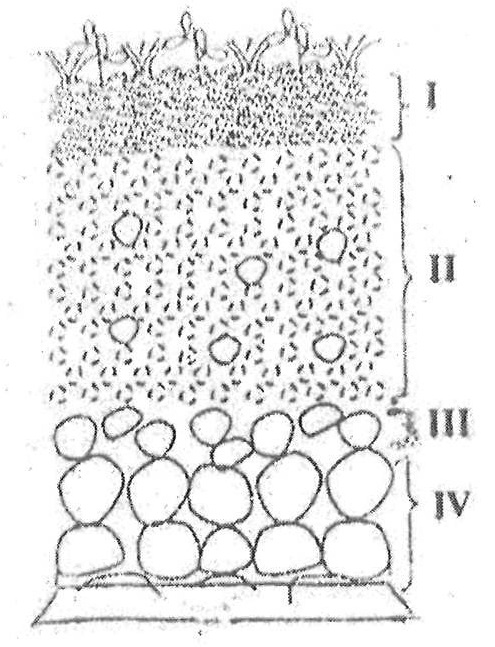Kuulchat

Online Education Resource Portal, its all freeBECE SCIENCE 2018

#### OBJECTIVE TEST

1.

The space occupied by matter is its

A.

area.

B.

mass.

C.

volume.

D.

weight.

2.

Which of the following structures contain cellulose?

A.

Cell wall

B.

Cell membrane

C.

Chloroplast

D.

Mitochondrion

3.

Which of the following activities are cultural practices used in tomato cultivation?

I. Pest control

II. Picking out

III. Fertilizer application

A.

I and II only

B.

I and III only

C.

II and III only

D.

I, II and III

4.

The farming system that makes effective use of available soil nutrients in a growing season is

A.

crop rotation.

B.

land rotation.

C.

mixed cropping.

D.

mixed farming.

5.

Which of the following members in a food chain will receive the least energy?

A.

Lizard

B.

Grass

C.

Grasshopper

D.

Hawk

6.

Digestion of fats and oils in humans starts in the

A.

colon.

B.

gullet.

C.

mouth.

D.

duodenum.

7.

What is the chemical symbol for silver?

A.

Ag

B.

Au

C.

Si

D.

S

8.

The process by which gas changes to liquid is known as

A.

melting.

B.

evaporation.

C.

condensation.

D.

boiling.

9.

Which of the following substances is acidic?

A.

Wood ash

B.

Salt-petre

C.

Bee strings

D.

Bicarbonate of soda

10.

The basic unit of life is the

A.

cell.

B.

nucleus.

C.

organ.

D.

tissue.

11.

Useful materials in food that provide growth and repair of worn out tissues are called

A.

carbohydrates.

B.

vitamins.

C.

proteins.

D.

fats.

12.

In human reproduction, the sperm fertilizes the female egg to form the

A.

zygote.

B.

follicle.

C.

ovary.

D.

ovum.

13.

Use the information below to answer the question that follow.

A solid X reacted with a liquid Y to form a salt and water only. A portion of liquid Y turned blue litmus paper red. Solid X did not dissolve when a portion of it was placed in water and stirred.

Solid X could be said to be

A.

an acid.

B.

an alkali.

C.

a base.

D.

a salt.

14.

Use the information below to answer the question that follow.

A solid X reacted with a liquid Y to form a salt and water only. A portion of liquid Y turned blue litmus paper red. Solid X did not dissolve when a portion of it was placed in water and stirred.

Liquid Y is

A.

an acid.

B.

an alkali.

C.

a base.

D.

a metal.

15.

Use the information below to answer the question that follow.

A solid X reacted with a liquid Y to form a salt and water only. A portion of liquid Y turned blue litmus paper red. Solid X did not dissolve when a portion of it was placed in water and stirred.

If red litmus paper is placed in liquid Y, the colour of the paper will

A.

remain the same.

B.

change to blue.

C.

change to purple.

D.

become brighter.

16.

The type of soil which become water-logged after rainfall is made up of a large amount of

A.

silt.

B.

sand.

C.

gravel.

D.

clay.

17.

Ringworm is a disease which attacks the

A.

skin.

B.

lungs.

C.

liver.

D.

kidneys.

18.

The most appropriate to adopt when growing vegetables in the dry season is

A.

watering.

B.

transplanting.

C.

pest control.

D.

fertilizer application.

19.

Which of the following organisms improves soil fertility?

A.

Caterpillar

B.

Millipede

C.

Nematode

D.

Rat

20.

The introduction of natural enemies to control pests on a field is a

A.

physical control method.

B.

cultural control method.

C.

chemical control method.

D.

biological control method.

21.

A body has a density of 30gcm-3 and a volume of 6cm3. Determine the mass of the body.

A.

180.0g

B.

24.0g

C.

5.0g

D.

0.2g

22.

The force which tends to pull an object in a circular motion towards the centre of the circle is called

A.

centripetal force.

B.

electrostatic force.

C.

gravitational force.

D.

magnetic force.

23.

The swollen shoot disease of cocoa is caused by

A.

fungus.

B.

virus.

C.

bacteria.

D.

protozoa.

24.

The instrument used to measure atmospheric pressure is called

A.

a thermometer.

B.

a force meter.

C.

a barometer.

D.

an anemometer.

25.

The systematic name of NO2 is

A.

nitrogen oxide.

B.

nitrogen (i) oxide.

C.

nitrogen (ii) oxide.

D.

nitrogen (iv) oxide.

26.

Which of the following simple machine is a second class lever?

A.

Bottle top opener

B.

Claw hammer

C.

Fishing rod

D.

Pair of pliers

27.

A cylinder metal has radius 7cm and height 2cm. Determine the volume of the cylinder.

[Take π = 3.143]

A.

44cm3

B.

107cm3

C.

308cm3

D.

1078cm3

28.

Which of the following statements are true of compounds?

I. They are homogenous

II. Their constituents are present in fixed ration by mass

III. Their constituents keep their individual properties

A.

I and II only

B.

I and III only

C.

II and III only

D.

I, II and III

29.

Which of the following factors contribute to early parenthood?

I. Illiteracy

II. Poverty

III. Peer pressure

IV. Lack of parental care

A.

I and II only

B.

II and III only

C.

III and IV only

D.

I, II, III and IV

30.

Which of the following statements about the negative use of Science and Technology is correct? It

A.

promotes good health.

B.

promotes easy communication.

C.

enable humans to use less energy in working.

D.

creates things that are dangerous to the environment.

31.

Which of the following nutrients is need by plants in small quantities?

A.

Calcium

B.

Iron

C.

Potassium

D.

Magnesium

32.

Which of the following statements about shadow formation is correct? It shows that light rays can

A.

be diffused.

B.

travel in a straight line.

C.

be refracted.

D.

be reflected.

33.

The colour of the neutral wire in an electrical circuit is

A.

blue.

B.

brown.

C.

green.

D.

yellow.

34.

A metal displaces 5.0cm3 of water when it is totally immersed. If the mass of the metal is 35.0g, calculate its density.

A.

7.0cm-3

B.

30.00cm-3

C.

40.00cm-3

D.

175.00cm-3

35.

Which of the following physical properties of water are correct? Water

I. is a colourless liquid.

II. is a universal solvent.

III. boils at 100°C at standard atmosphere pressure.

IV. has a density of 5kgcm-3 at 4°C.

A.

I and II only

B.

II and III only

C.

I, II and III only

D.

II, III and IV only

36.

The relative proportions of the different particle sizes in a soil sample refer to soil

A.

temperature.

B.

texture.

C.

structure.

D.

porosity.

37.

Which of the following chemical equations is balanced?

A.

K + O2→K2O

B.

Mg + O2→MgO

C.

Na + Cl2→NaCl

D.

H2 + Cl2→2HCl

38.

Heat is transferred along an iron bar by

A.

absorption.

B.

conduction.

C.

convection.

D.

39.

The second large in the life cycle of a mosquito is the

A.

egg.

B.

imago.

C.

larva.

D.

pupa.

40.

The function of the platelets in the circulatory system of humans is to

A.

transport oxygen.

B.

transport carbon dioxide.

C.

defend the body.

D.

cloth the blood.

#### THEORY QUESTIONS

1.

The diagram below is an illustration of a fish.

Study the diagram carefully and answer the questions that follow.(a) (i) Identify the fish.

(ii) Name each of the parts labeled I, II, III and V.

(iii) Name that habitat of the fish.

(iv) Explain how each of the parts labelled III and VI enables the fish adapt to its habitat.

(b) The diagram below is an illustration of a section through the soil.

Study the diagram carefully and answer the questions that follow.(i) What does the diagram represent?

(ii) Name each of the parts labelled I, II, III, and IV.

(iii) Which part of the diagram:

(α) is the richest in humus?

(β) is the habitat for soil organisms?

(γ) undergoes weathering?

(iv) What is the effect of heavy rainfall on the part labelled I?

(c) The diagram below is an illustration of an electrical circuit.

Study the diagram carefully and answer the questions that follow.(i) Name each of the parts labelled I, II, IV, VI.

(ii) State the energy transformation that takes place in:

(α) I

(β) IV

(iii) State the S.I units of the quantity measured by each of the parts labelled:

(α) III

(β) V

(iv) State the function of the part labelled VI.

(d) In an experiment, equal volumes and equal concentrations of dilute hydrochloric acid and dilute sodium hydroxide solutions were each placed in different test tubes.

I. Both red and blue litmus papers were dipped into each of the solutions in turns.

II. Equal volumes of the solutions were mixed to obtain a third solution.

III. Both red and blue litmus papers were dipped into the third solution.

Use the information provided to answer the following questions.

(i) Explain briefly how you can identify each of the solutions

(α) Hydrochloric acid

(β) Sodium hydroxide

(ii) State the types of reaction that occurred when the two solutions were mixed.

(iii) What type of solution was formed when the reaction stated in (ii) occurred?

(iv) State what would be observed when both red and blue litmus papers were dipped into the third solution.

(v) Explain how the solid portion of the solution named in (iii) could be obtained.

Show Solution
2.

(i) What is an ion?

(ii) State two methods of softening hard water.

(b) (i) Differentiate between pest and parasites as used in a agriculture.

(ii) Give an example each of a:

(α) pest;

(β) parasites.

(c) (i) What is work?

(ii) A force of 10N causes a body to move a distance of 5.2m in the direction of the force. Calculate the work done.

(d) Name two diseases associated with the circulatory system of humans.

Show Solution
3.

(a) (i) What is malnutrition?

(ii) State one symptom each of the following deficiency disease:

(α) scurvy;

(β) rickets.

(b) Draw the potassium atom and show the distribution of electrons in its shells.

[K= 19]

(c) (i) Define potential energy.

(ii) An object of mass 10kg is moving at a velocity of 2ms-1.

Calculate the kinetic energy of the object.

(d) State one example each of:

(i) macro nutrients;

(ii) micro nutrients.

Show Solution
4.

(a) (i) Explain the term hazard.

(ii) List two safety precautions against hazards in the teaching and learning of science.

(b) In a tabular form state three differences between osmosis and diffusion.

(c) (i) What is weather?

(ii) State two differences between weather and season.

(d) (i) What is a fertile soil?

(ii) State two factors that cause loss of soil fertility.

Show Solution
5.

(a) (i) What is a magnetic field?

(ii) Name two methods of making magnets.

(b) Explain briefly the term teenage pregnancy.

(c) Write the formula for each of the following compounds:

(i) calcium chloride;

(ii) copper (I) oxide;

(iii) nitrogen (IV) oxide;

(iv) ammonia.

(d) (i) List three physical properties of soil.

(ii) What is the texture of clayey soil?

Show Solution
6.

(a) (i) What are derived quantities?

(ii) State the S.I units of the following quantities:

(α) area;

(β) volume.

(b) (i) State two factors necessary for photosynthesis.

(ii) Explain the functions of each of the factors stated in (i).

(c) Explain each of the following terms:

(i) Soft water;

(ii) Hard water.

(d) State three reasons why some seeds are nursed.

Show Solution